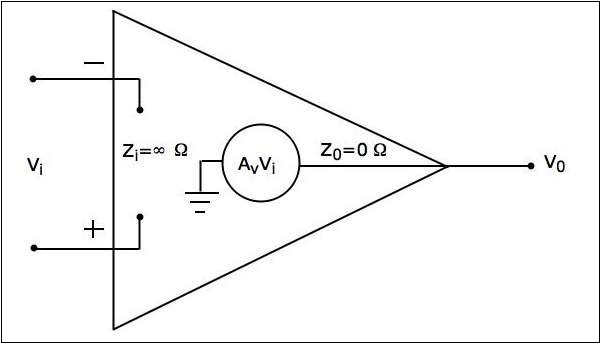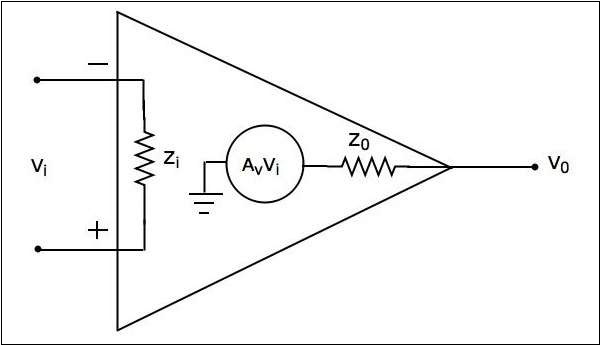# Basics Of Operational Amplifier

Advertisements

Operational Amplifier, also called as an Op-Amp, is an integrated circuit, which can be used to perform various linear, non-linear, and mathematical operations. An op-amp is a direct coupled high gain amplifier. You can operate op-amp both with AC and DC signals. This chapter discusses the characteristics and types of op-amps.

## Construction of Operational Amplifier

An op-amp consists of differential amplifier(s), a level translator and an output stage. A differential amplifier is present at the input stage of an op-amp and hence an op-amp consists of two input terminals. One of those terminals is called as the inverting terminal and the other one is called as the non-inverting terminal. The terminals are named based on the phase relationship between their respective inputs and outputs.

### Characteristics of Operational Amplifier

The important characteristics or parameters of an operational amplifier are as follows −

• Open loop voltage gain
• Output offset voltage
• Common Mode Rejection Ratio
• Slew Rate

This section discusses these characteristics in detail as given below −

### Open loop voltage gain

The open loop voltage gain of an op-amp is its differential gain without any feedback path.

Mathematically, the open loop voltage gain of an op-amp is represented as −

$$A_{v}= \frac{v_0}{v_1-v_2}$$

### Output offset voltage

The voltage present at the output of an op-amp when its differential input voltage is zero is called as output offset voltage.

### Common Mode Rejection Ratio

Common Mode Rejection Ratio (CMRR) of an op-amp is defined as the ratio of the closed loop differential gain, $A_{d}$ and the common mode gain, $A_{c}$.

Mathematically, CMRR can be represented as −

$$CMRR=\frac{A_{d}}{A_{c}}$$

Note that the common mode gain, $A_{c}$ of an op-amp is the ratio of the common mode output voltage and the common mode input voltage.

### Slew Rate

Slew rate of an op-amp is defined as the maximum rate of change of the output voltage due to a step input voltage.

Mathematically, slew rate (SR) can be represented as −

$$SR=Maximum\:of\:\frac{\text{d}V_{0}}{\text{d}t}$$

Where, $V_{0}$ is the output voltage. In general, slew rate is measured in either $V/\mu\:Sec$ or $V/m\:Sec$.

## Types of Operational Amplifiers

An op-amp is represented with a triangle symbol having two inputs and one output.

Op-amps are of two types: Ideal Op-Amp and Practical Op-Amp.

They are discussed in detail as given below −

### Ideal Op-Amp

An ideal op-amp exists only in theory, and does not exist practically. The equivalent circuit of an ideal op-amp is shown in the figure given below −An ideal op-amp exhibits the following characteristics −

• Input impedance $Z_{i}=\infty\Omega$

• Output impedance $Z_{0}=0\Omega$

• Open loop voltage gaine $A_{v}=\infty$

• If (the differential) input voltage $V_{i}=0V$, then the output voltage will be $V_{0}=0V$

• Bandwidth is infinity. It means, an ideal op-amp will amplify the signals of any frequency without any attenuation.

• Common Mode Rejection Ratio (CMRR) is infinity.

• Slew Rate (SR) is infinity. It means, the ideal op-amp will produce a change in the output instantly in response to an input step voltage.

### Practical Op-Amp

Practically, op-amps are not ideal and deviate from their ideal characteristics because of some imperfections during manufacturing. The equivalent circuit of a practical op-amp is shown in the following figure −A practical op-amp exhibits the following characteristics −

• Input impedance, $Z_{i}$ in the order of Mega ohms.

• Output impedance, $Z_{0}$ in the order of few ohms..

• Open loop voltage gain, $A_{v}$ will be high.

When you choose a practical op-amp, you should check whether it satisfies the following conditions −

• Input impedance, $Z_{i}$ should be as high as possible.

• Output impedance, $Z_{0}$ should be as low as possible.

• Open loop voltage gain, $A_{v}$ should be as high as possible.

• Output offset voltage should be as low as possible.

• The operating Bandwidth should be as high as possible.

• CMRR should be as high as possible.

• Slew rate should be as high as possible.

Note − IC 741 op-amp is the most popular and practical op-amp.

Advertisements